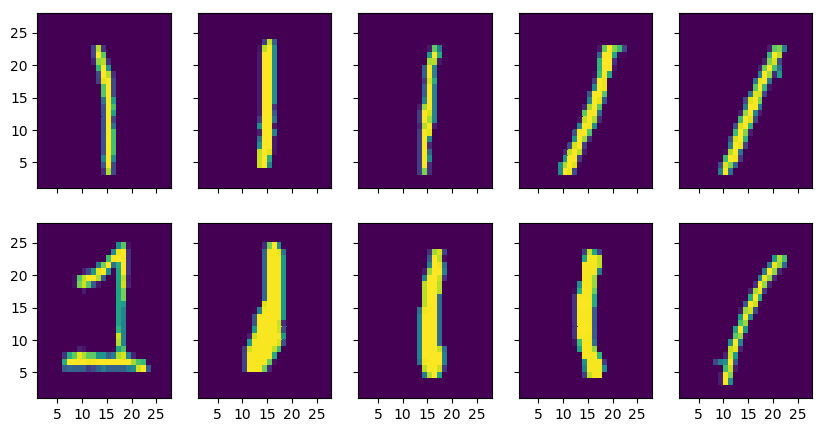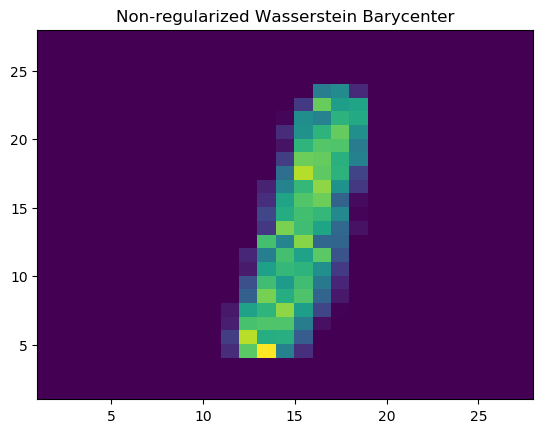# Implementing Wasserstein Barycenter problem using JuMP in Julia.¶

The goal of this notebook is to implement a model to calculate the Wasserstein barycenter in Julia with JuMP and then solve it using MosekTools. For additional info about the data used, theoretical explanation of the calculation of barycenters, references and for more insight in construction of the model, please consult the corresponding Python notebook. Data files can be found in http://yann.lecun.com/exdb/mnist/.

In :
using LinearAlgebra
using Plots
pyplot()

using JuMP

using MosekTools

In :
#Define the number of images for the barycenter calculation.
n = 20

#Read the images from the file.
function read_idx(filename)
f = open(filename,"r")
data_layout = zeros(UInt8,4)
readbytes!(f,data_layout,4)
data_zero = reinterpret(UInt16,data_layout[1:2])
data_type,data_dimensions = reinterpret(UInt8,data_layout[3:4])
data_shape = Int32[]
for i = 1:data_layout
s = zeros(UInt8,4)
readbytes!(f,s,4)
s = map(hton,reinterpret(Int32,s))
push!(data_shape,s)
end
idx_data = zeros(UInt8,cumprod(data_shape)[length(data_shape)])
read!(f,idx_data)
idx_data = reshape(idx_data,Tuple(reverse(data_shape)))
return(idx_data)
end

data = read_idx("train-images-idx3-ubyte")
labels = read_idx("train-labels-idx1-ubyte")

#Select the images.
mask = labels .== 1
train_ones = data[:,:,mask]
train = train_ones[:,:,1:n]

x = [i for i=1:28]
y = reverse(x)
f,ax = PyPlot.plt.subplots(2,5,sharey=true,sharex=true,figsize=(10,5))
PyPlot.plt.xticks([5,10,15,20,25])

for i = 1:10
rand_pick = rand(1:size(train_ones))
ax[i].pcolormesh(x,y,transpose(train_ones[:,:,rand_pick]))
end# Barycenters using JuMP¶

In :
function single_pmf(data)
#Takes a list of images and extracts the probability mass function.
v = vec(data[:,:,1])
v = v./cumsum(v)[length(v)]
for im_k in 2:size(data)
image = data[:,:,im_k]
arr = vec(image)
v_size = size(arr)
v = hcat(v, arr./cumsum(arr)[length(arr)])
end
return v,size(v)
end

function ms_distance(m,n)
#Squared Euclidean distance calculation between the pixels.
d = ones(m,m)
coor_I = []
for c_i in 1:n
append!(coor_I,ones(Int,n).*c_i)
end
coor_J = repeat(1:n,n)
coor = hcat(coor_I,coor_J)
for i in 1:m
for j in 1:m
d[i,j] = norm(coor[i,:]-coor[j,:]).^2
end
end
return d
end

function wasserstein_barycenter(data)
M= direct_model(Mosek.Optimizer())

if length(size(data))==3
K = size(data)
else
K = 1
end
v,N = single_pmf(data)
d = ms_distance(N,size(data))

#Define indices
M_i = 1:N
M_j = 1:N
M_k = 1:K

#Adding variables
M_pi = @variable(M, M_pi[i = M_i, j = M_j, k = M_k] >= 0.0)
M_mu = @variable(M, M_mu[i = M_i] >= 0.0)

#Adding constraints
@constraint(M, c3_expr[k = M_k, j = M_j], sum(M_pi[:,j,k]) == v[j,k])
@constraint(M, c2_expr[k = M_k, i = M_i], sum(M_pi[i,:,k]) == M_mu[i])

#Objective
W_obj = @objective(M, Min, sum(d[i,j]*M_pi[i,j,k] for i=M_i,j=M_j,k=M_k)/K)

return M,M_mu
end

Out:
wasserstein_barycenter (generic function with 1 method)
In :
function run_model(data)
@time begin
M,M_mu = wasserstein_barycenter(data)
optimize!(M)
end
println("Solution status = ",termination_status(M))
println("Primal objective value = ",objective_value(M))
mu_level = value.(M_mu)
return mu_level
end

function show_barycenter(bary_center)
bary_center = reshape(bary_center,(28,28))
x = [i for i=1:28]
y = reverse(x)
PyPlot.plt.pcolormesh(x,y,transpose(bary_center))
PyPlot.plt.title("Non-regularized Wasserstein Barycenter")
PyPlot.plt.show()
end

Out:
show_barycenter (generic function with 1 method)
In :
bary_center = run_model(train)
println("******")

Problem
Name                   :
Objective sense        : min
Type                   : LO (linear optimization problem)
Constraints            : 31360
Cones                  : 0
Scalar variables       : 12293904
Matrix variables       : 0
Integer variables      : 0

Optimizer started.
Presolve started.
Linear dependency checker started.
Linear dependency checker terminated.
Eliminator started.
Freed constraints in eliminator : 0
Eliminator terminated.
Eliminator - tries                  : 1                 time                   : 0.00
Lin. dep.  - tries                  : 1                 time                   : 1.00
Lin. dep.  - number                 : 19
Presolve terminated. Time: 24.73
GP based matrix reordering started.
GP based matrix reordering terminated.
Problem
Name                   :
Objective sense        : min
Type                   : LO (linear optimization problem)
Constraints            : 31360
Cones                  : 0
Scalar variables       : 12293904
Matrix variables       : 0
Integer variables      : 0

Optimizer  - threads                : 20
Optimizer  - solved problem         : the primal
Optimizer  - Constraints            : 17440
Optimizer  - Cones                  : 0
Optimizer  - Scalar variables       : 1395520           conic                  : 0
Optimizer  - Semi-definite variables: 0                 scalarized             : 0
Factor     - setup time             : 25.48             dense det. time        : 1.58
Factor     - ML order time          : 0.06              GP order time          : 20.69
Factor     - nonzeros before factor : 1.55e+06          after factor           : 1.44e+07
Factor     - dense dim.             : 0                 flops                  : 1.64e+10
Factor     - GP saved nzs           : 1.75e+06          GP saved flops         : 3.15e+09
ITE PFEAS    DFEAS    GFEAS    PRSTATUS   POBJ              DOBJ              MU       TIME
0   5.5e+03  3.2e+02  8.3e+07  0.00e+00   1.182613040e+07   0.000000000e+00   4.9e+01  53.40
1   6.8e-01  4.0e-02  1.0e+04  -1.00e+00  1.120231124e+07   -1.638527155e+05  6.1e-03  54.77
2   5.8e-02  3.4e-03  8.8e+02  2.61e+01   1.558624793e+04   -2.985004056e+03  5.2e-04  56.57
3   4.5e-02  2.6e-03  6.8e+02  1.08e+01   3.256531610e+03   -6.820367822e+02  4.0e-04  57.82
4   4.2e-02  2.5e-03  6.4e+02  5.25e+00   2.407863337e+03   -4.995626509e+02  3.8e-04  58.96
5   3.8e-02  2.2e-03  5.7e+02  4.40e+00   1.571023934e+03   -3.171235831e+02  3.4e-04  60.16
6   3.3e-02  1.9e-03  5.0e+02  3.48e+00   1.104556063e+03   -2.125212676e+02  3.0e-04  61.35
7   2.9e-02  1.7e-03  4.3e+02  2.91e+00   7.928892890e+02   -1.415543156e+02  2.6e-04  62.37
8   1.2e-02  7.1e-04  1.8e+02  2.47e+00   2.252273636e+02   -1.976506734e+01  1.1e-04  63.67
9   5.2e-03  3.1e-04  7.9e+01  1.44e+00   9.137869757e+01   -2.802281268e+00  4.7e-05  64.92
10  1.2e-03  6.9e-05  1.8e+01  1.16e+00   2.223918686e+01   2.047776185e+00   1.1e-05  67.16
11  7.7e-04  4.5e-05  1.2e+01  1.04e+00   1.552926524e+01   2.419699999e+00   6.9e-06  68.42
12  3.9e-04  2.3e-05  5.9e+00  1.02e+00   9.413697273e+00   2.768959100e+00   3.5e-06  69.85
13  1.3e-04  8.1e-06  2.0e+00  1.01e+00   5.274785616e+00   3.000446303e+00   1.2e-06  71.81
14  5.5e-05  3.3e-06  8.2e-01  1.00e+00   3.991063103e+00   3.070294704e+00   4.9e-07  73.18
15  2.5e-05  1.5e-06  3.8e-01  1.00e+00   3.515580593e+00   3.095346906e+00   2.2e-07  74.61
16  1.2e-05  7.0e-07  1.8e-01  1.00e+00   3.304330090e+00   3.106260422e+00   1.1e-07  76.16
17  7.1e-06  4.3e-07  1.1e-01  1.00e+00   3.229749859e+00   3.109863797e+00   6.4e-08  77.29
18  3.1e-06  2.4e-07  4.8e-02  1.00e+00   3.165710662e+00   3.112133239e+00   2.8e-08  78.38
19  1.2e-06  9.4e-08  1.9e-02  1.00e+00   3.135044854e+00   3.113925074e+00   1.1e-08  79.69
20  2.5e-07  1.9e-08  3.9e-03  1.00e+00   3.119045127e+00   3.114732420e+00   2.3e-09  80.96
21  1.2e-09  9.1e-11  1.8e-05  1.00e+00   3.114878903e+00   3.114858640e+00   1.1e-11  82.84
22  3.7e-12  2.5e-13  4.7e-08  1.00e+00   3.114858824e+00   3.114858771e+00   2.8e-14  83.82
23  1.8e-13  5.7e-14  4.2e-13  1.00e+00   3.114858772e+00   3.114858772e+00   2.9e-18  84.82
Basis identification started.
Primal basis identification phase started.
Primal basis identification phase terminated. Time: 0.13
Dual basis identification phase started.
Dual basis identification phase terminated. Time: 0.11
Basis identification terminated. Time: 1.09
Optimizer terminated. Time: 101.45

246.621270 seconds (373.68 M allocations: 35.441 GiB, 28.30% gc time)
Solution status = OPTIMAL
Primal objective value = 3.1148587717952356
******

In :
show_barycenter(bary_center)This work is licensed under a Creative Commons Attribution 4.0 International License. The MOSEK logo and name are trademarks of Mosek ApS. The code is provided as-is. Compatibility with future release of MOSEK or the Fusion API are not guaranteed. For more information contact our support.

In [ ]: